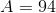## Example Questions

### Example Question #23 : Sat Mathematics

An equilateral triangle with side lengthhas one of its vertices at the center of a regular hexagon, and the side opposite that vertex is one of the sides of the hexagon. What is the hexagon's area?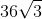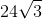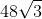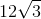Explanation:

Because it can be split into two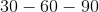triangles, the area of an equilateral triangle can be expressed as...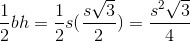With that in mind, the equilateral triangle in question has area of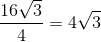.

Now consider that a regular hexagon can be split into six congruent equilateral triangles with a vertex at the center and the side opposite the center as one of the hexagon's sides (a handy way of finding a hexagon's area if you can't use the regular polygon formula requiring an apothem.) Knowing that, our answer is.

### Example Question #1 : How To Find The Area Of A Hexagon

Calculate the approximate area a regular hexagon with the following side length: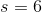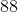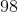Cannot be determined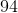Explanation:

# How do you find the area of a hexagon?

There are several ways to find the area of a hexagon.

1. In a regular hexagon, split the figure into triangles.
2. Find the area of one triangle.
3. Multiply this value by six.

Alternatively, the area can be found by calculating one-half of the side length times the apothem.

## Regular hexagons:

Regular hexagons are interesting polygons. Hexagons are six sided figures and possess the following shape:

In a regular hexagon, all sides equal the same length and all interior angles have the same measure; therefore, we can write the following expression.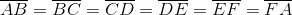One of the easiest methods that can be used to find the area of a polygon is to split the figure into triangles. Let's start by splitting the hexagon into six triangles.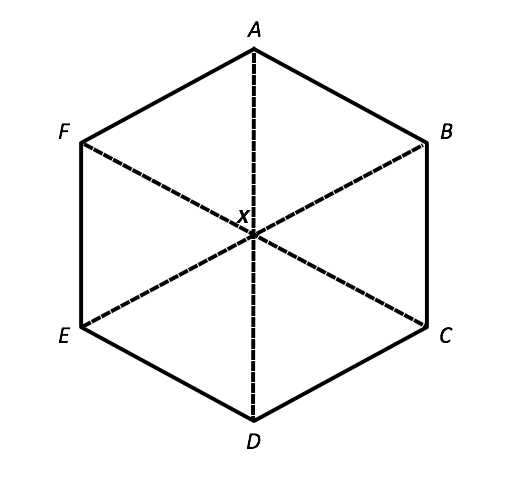In this figure, the center point,, is equidistant from all of the vertices. As a result, the six dotted lines within the hexagon are the same length. Likewise, all of the triangles within the hexagon are congruent by the side-side-side rule: each of the triangle's share two sides inside the hexagon as well as a base side that makes up the perimeter of the hexagon. In a similar fashion, each of the triangles have the same angles. There are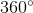in a circle and the hexagon in our image has separated it into six equal parts; therefore, we can write the following: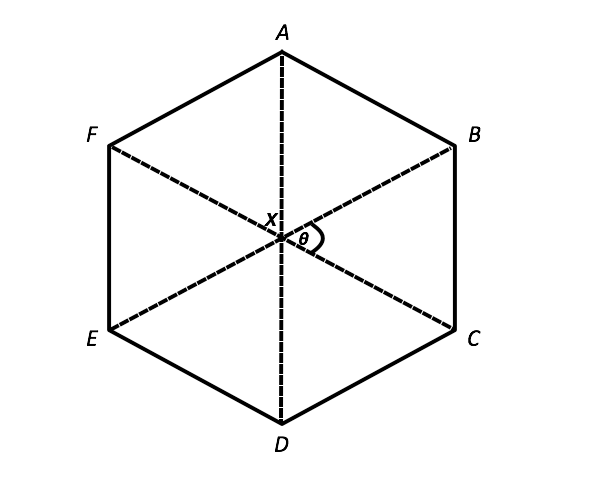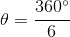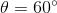We also know the following: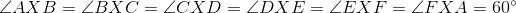Now, let's look at each of the triangles in the hexagon. We know that each triangle has two two sides that are equal; therefore, each of the base angles of each triangle must be the same. We know that a triangle hasand we can solve for the two base angles of each triangle using this information.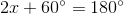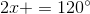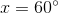Each angle in the triangle equals. We now know that all the triangles are congruent and equilateral: each triangle has three equal side lengths and three equal angles. Now, we can use this vital information to solve for the hexagon's area. If we find the area of one of the triangles, then we can multiply it by six in order to calculate the area of the entire figure. Let's start by analyzing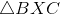. If we draw, an altitude through the triangle, then we find that we create two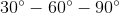triangles.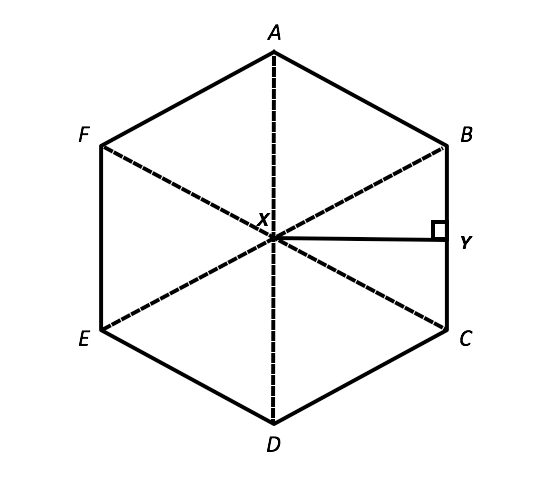Let's solve for the length of this triangle. Remember that intriangles, triangles possess side lengths in the following ratio: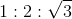Now, we can analyzeusing the a substitute variable for side length,.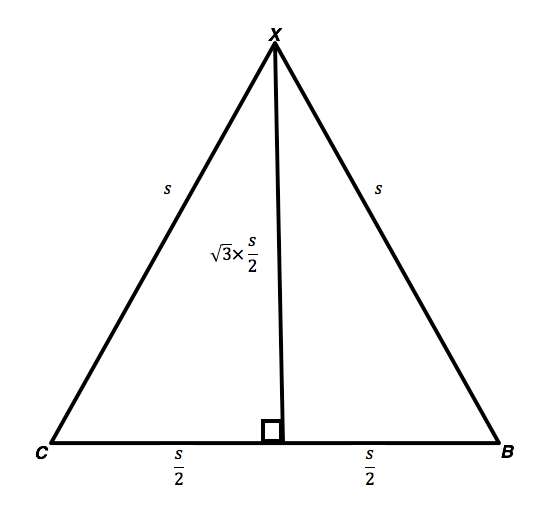We know the measure of both the base and height ofand we can solve for its area.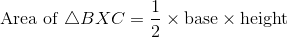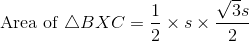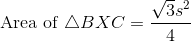Now, we need to multiply this by six in order to find the area of the entire hexagon.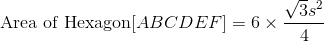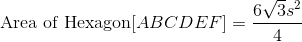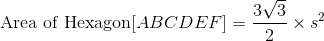We have solved for the area of a regular hexagon with side length,. If we know the side length of a regular hexagon, then we can solve for the area.

If we are not given a regular hexagon, then we an solve for the area of the hexagon by using the side length(i.e.) and apothem (i.e.), which is the length of a line drawn from the center of the polygon to the right angle of any side. This is denoted by the variablein the following figure: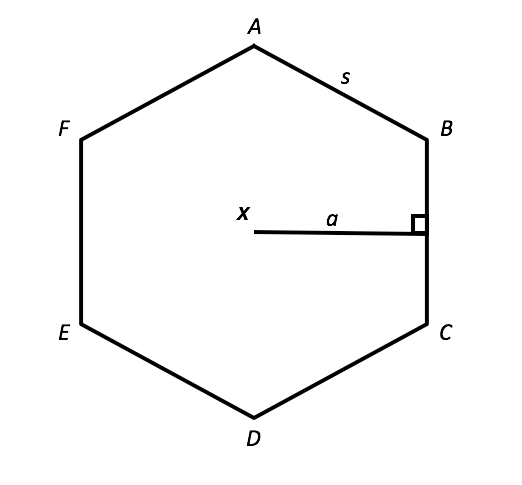## Alternative method:

If we are given the variablesand, then we can solve for the area of the hexagon through the following formula: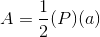In this equation,is the area,is the perimeter, andis the apothem. We must calculate the perimeter using the side length and the equation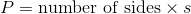, whereis the side length.

## Solution:

In the given problem we know that the side length of a regular hexagon is the following:Let's substitute this value into the area formula for a regular hexagon and solve.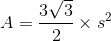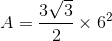Simplify.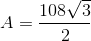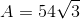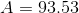Round the answer to the nearest whole number.